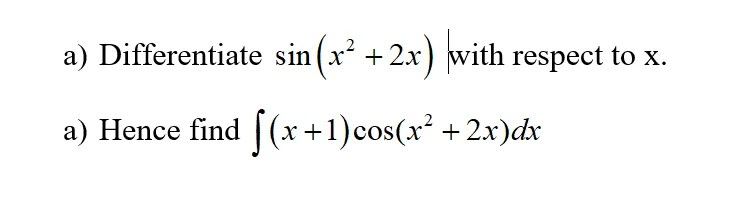# A-Math – Differentiation

Home/A-Math - Differentiation

## Additional Math – Differentiation – Sin 2x and 2 lnx

Use Quotient Rule $latex \displaystyle \frac{{dy}}{{dx}}=\frac{{v\frac{{du}}{{dx}}-u\frac{{dv}}{{dx}}}}{{{{v}^{2}}}}$ Differentiate the variables separately before inserting into the rule, there is less chance of [...]

By |2020-02-24T22:10:53+08:00February 24th, 2020|A-Math - Differentiation|

## Additional Math – Differentiation – Equations with cot x and cosec x

$latex \displaystyle y={{\cot }^{2}}x-5+\cos ecx$ It is difficult to differentiate cot x and cosec x directly. You need to change [...]

By |2018-12-23T11:26:43+08:00December 23rd, 2018|A-Math - Differentiation, A-Math - Trigonometry|

## Additional Math – Coordinate Geometry and Differentiation – Determine the unknown value k

To find the value of k, you need to first find the gradient of the tangent of the curve. Then [...]

By |2018-09-07T13:46:57+08:00September 7th, 2018|A-Math - Coordinate Geometry, A-Math - Differentiation|

## Additional Math – Differentiate – Natural Log (ln) and Cube Root

$latex \displaystyle \text{y = ln}\sqrt{{\frac{{3x-1}}{{x+5}}}}$ Before you Differentiate, simplify the expression by changing the cube root to index form followed [...]

By |2018-03-27T10:37:10+08:00March 27th, 2018|A-Math - Differentiation|

$latex \displaystyle y=\ \ln \left( {\frac{{{{e}^{{{{x}^{2}}}}}}}{{\cos (1-x)}}} \right)$ Before you Differentiate, simplify the expression using the Laws of Logarithm   $latex [...] By |2018-03-27T10:45:41+08:00March 22nd, 2018|A-Math - Differentiation| ## Additional Math – Differentiate – Trigo (Sine) and Natural Log (ln)$latex \displaystyle y=\ \text{ln}\left( {\frac{{\sin x-1}}{{3x+2}}} \right)\$ Use the Law of Logarithm Rule lg (x/y) = lg x - lg [...]

By |2018-03-27T10:47:39+08:00March 9th, 2018|A-Math - Differentiation|

## Additional Math – 2nd order Differentiation and Integration – Find the equation of the curve

Sun 12 noon Additional Math Tuition Class at Woodlands- Differentiation of gradient to find the equation of curve. [...]

By |2017-11-15T13:21:05+08:00August 22nd, 2017|A-Math - Differentiation, A-Math - Integration|
•Additional Math – Integration – Integration of Product
Gallery

## Additional Math – Integration – Integration of Product

Sunday Tuition at Woodlands at 2.00pm - Differentiation and Integration of Trigonometry Differentiation and Integration of Trigonometric Functions [...]

By |2017-11-17T07:47:09+08:00June 7th, 2017|A-Math - Differentiation, A-Math - Integration|